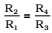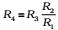# Wheatstone Bridge – Working Principle, Formula, Derivation

0
155

## What is Wheatstone Bridge?

Wheatstone bridge, also known as the resistance bridge, calculates the unknown resistance by balancing two legs of the bridge circuit. One leg includes the component of unknown resistance.

The Wheatstone Bridge Circuit comprises two known resistors, one unknown resistor and one variable resistor connected in the form of a bridge. This bridge is very reliable as it gives accurate measurements.

## Construction of Wheatstone Bridge

A Wheatstone bridge circuit consists of four arms, of which two arms consist of known resistances while the other two arms consist of an unknown resistance and a variable resistance. The circuit also consists of a galvanometer and an electromotive force source. The emf source is attached between points a and b while the galvanometer is connected between points c and d. The current that flows through the galvanometer depends on its potential difference.

## What is the Wheatstone Bridge Principle?

The Wheatstone bridge works on the principle of null deflection, i.e. the ratio of their resistances is equal, and no current flows through the circuit. Under normal conditions, the bridge is in an unbalanced condition where current flows through the galvanometer. The bridge is said to be balanced when no current flows through the galvanometer. This condition can be achieved by adjusting the known resistance and variable resistance.

It is an arrangement of four resistance used to determine one of this resistance quickly and accurately in terms of the remaining three resistances.The bridge has four resistors R1R2R3 and R4. Across one pair of diagonally opposite points (A and C in the figure) a source is connected. This (i.e., AC) is called the battery arm. Between the other two vertices, B and D, a galvanometer G (which is a device to detect currents) is connected. This line, shown as BD in the figure, is called the galvanometer arm.• When the Wheatstone bridge is balanced, the potential difference between the points B and D is zero.
• The Wheatstone bridge is most sensitive when the resistances in the four arms are of the same order.
• Wheatstone bridge method is not suitable for the measurement of very low and very high resistance.
• If the bridge is balanced, then on interchanging the positions of the galvanometer and the battery there is no effect on the balance of the bridge. That is why the arms BD and Ac are called conjugate arms of the bridge.

## Wheatstone Bridge Formula

R=PS/Q

Where:

• R is the unknown resistance
• S is the standard arm of the bridge
• P and Q is the ratio of the arm of the bridge

## Wheatstone Bridge Application

• The Wheatstone bridge is used for the precise measurement of low resistance.
• Wheatstone bridge and an operational amplifier are used to measure physical parameters such as temperature, light, and strain.
• Quantities such as impedance, inductance, and capacitance can be measured using variations on the Wheatstone bridge.

## Wheatstone Bridge Limitations

• For low resistance measurement, the resistance of the leads and contacts becomes significant and introduces an error.
• For high resistance measurement, the measurement presented by the bridge is so large that the galvanometer is insensitive to imbalance.
• The other drawback is the resistance change due to the current’s heating effect through the resistance. Excessive current may even cause a permanent change in the value of resistance.